# Solving for any variable...Rearranging Equations - Practice Problems

Practice rearranging equations below using the "rules" that you have just learned. Answers are provided (but try doing them on your own before peeking!) You can download a sheet with all the questions (Acrobat (PDF) 127kB Jul25 09) so you can print them out to try on your own.

## Velocity, distance and time

Many problems in the geosciences deal with the concept of velocity, from stream flow to plate motion. Being able to manipulate the equation for velocity (v = d/t) gives you a powerful tool for understanding the world around you. And there are two other equations that you can get by rearranging the equation that you probably already know for velocity (also rate)!

Problem 1:Generally, we know the equation for velocity (a rate) to be: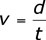Where v = velocity, d = distance and t = time.

This equation can be rearranged so that you have an equation for distance (d) and time (t).
1. Rearrange the velocity equation to create an equation for distance (d).
2. Rearrange the velocity equation to create an equation for time (t).
Use the equations that you manipulated above to solve the following problems: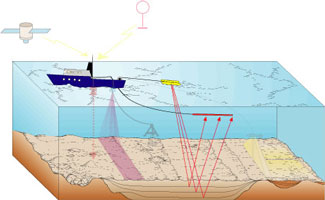Problem 2:A wave traveling downward from the surface of the ocean at 1.5 km/sec takes six seconds to reflect off the ocean floor. How deep is the ocean at that site?
Problem 3:Imagine that you are working with Ms. Homeowner to understand the groundwater flow in her area. She is particularly interested in an underground tank that is located 2.6 km from her home. You have measured the velocity of the groundwater to be 0.033 km/day. About how long will it take any contaminants leaking from the tank to reach Ms. Homeowner's well?

## Density

Density plays an important role in our understanding of the physical properties of Earth materials. The equation for density is similar to that for velocity and, as such, it can be manipulated so that you can solve for any of the variables involved. The next few problems utilize the equation for density: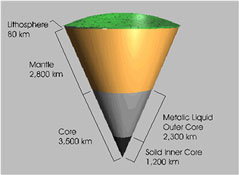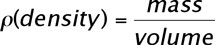which can be shortened to: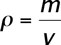Problem 1: Manipulate (rearrange) the density equation (above) to create an equation for mass.
Problem 2:Manipulate the density equation to create an equation for volume.
Use the equations that you manipulated above to solve the following problems:

Problem 3:
Continental crust has a density of about 2.75 g/cm3. What volume would 1000 g (1 kg) of continental crust occupy?
Problem 4:The outer core has a density of about 10.5 g/cm3. What volume would 1000 g (1 kg) of the outer core occupy?

## Isostasy

Isostasy is an important concept in the geosciences that is related to density. The concept of isostasy explains why continental crust sits so much higher than oceanic crust. It involves properties of an object like height and density. It also explains why only a portion of an iceberg is visible above water. The following problems involve an equation that is important to understanding the way that continental crust/oceanic crust behave on the Earth. If you need help visualizing the problem, click on the illustration below to see a larger version.

The equation for calculating the height of an object above the "fluid" in which it is floating is: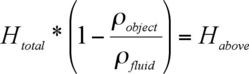where:

Htotal = total height of the object
ρobject = density of the object of interest (e.g., iceberg, continental crust, etc.)
ρfluid = density of the fluid in which the object is floating (e.g., seawater, mantle, etc.)
Habove = height of the object visible above the fluid.

Problem 1: Rearrange the equation above to solve for Htotal.

Problem 2: Use the equation that you generated in Problem 1 to calculate the thickness (Htotal) of continental crust if:

• the density of continental crust (ρobject) is 2.79 g/cm3,
• the density of the mantle (ρfluid) is 3.3 g/cm3,
• and, the average height of the continents above the mantle is 4.5 km (Habove).

Problem 3: Use the information from Problem 2 to calculate the thickness of crust that lies below the mantle (e.g., Hbelow).

## Next Steps

I think I've mastered manipulating equations! Let me try the quiz!
(If this is not how you feel, see the links below for more practice!)

## Still need more practice?

There are many web sites and books that walk you through the algebraic manipulation of equations. You can use these to get more practice with rearranging equations. You may need to go to the library to find some of these.